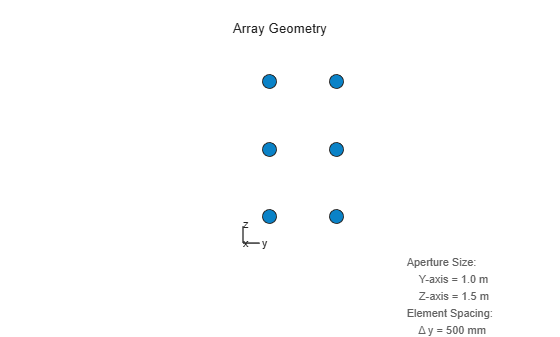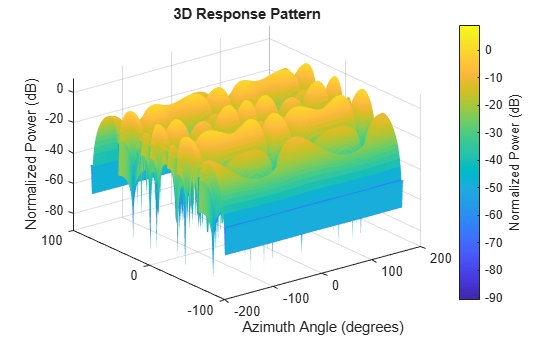Main Content

## Uniform Rectangular Array

### Support for Uniform Rectangular Arrays

You can implement a uniform rectangular array (URA) with `phased.URA`. Array elements are distributed in the yz-plane with the array look direction along the positive x-axis. When you use `phased.URA`, you must specify these aspects of the array:

• Sensor elements of the array

• Number of rows and the spacing between them

• Number of columns and the spacing between them

• Geometry of the planar lattice, which can be rectangular or triangular

### Uniform Rectangular Array of Isotropic Antenna Elements

This example shows you how to create a uniform rectangular array (URA) and obtain information about the element positions, the array response, and inter-element time delays. Then, simulate the reception of two sine waves coming from different directions. Both signals have a 1GHz carrier frequency.

Note: This example runs only in R2016b or later. If you are using an earlier release, replace each call to the function with the equivalent `step` syntax. For example, replace `myObject(x)` with `step(myObject,x)`.

Create the URA and obtain the element positions

Create and view a six-element URA with two elements along the y-axis and three elements along the z-axis. Use a rectangular lattice, with the default spacing of 0.5 meters along both the row and column dimensions of the array. Each element is an isotropic antenna element, which is the default element type for a URA.

```fc = 1e9; array = phased.URA([3,2]); viewArray(array)````pos = getElementPosition(array);`

The x-coordinate is zero for all elements of the array.

Compute the element delays

Calculate the element delays for signals arriving from +45° and -45° azimuth and 0° elevation.

```delay = phased.ElementDelay('SensorArray',array); ang = [45,-45]; tau = delay(ang);```

The first column of `tau` contains the element delays for the signal incident on the array from +45° azimuth. The second column contains the delays for the signal arriving from -45°. The delays are equal in magnitude but opposite in sign, as expected.

Compute the received signals

The following code simulates the reception of two sinusoidal waves arriving from far field sources. One signal is a 100-Hz sine wave arriving from 20° azimuth and 10° elevation. The second signal is a 300-Hz sine wave arriving from -30° azimuth and 5° elevation.

```t = linspace(0,1,1000); x1 = cos(2*pi*100*t)'; x2 = cos(2*pi*300*t)'; ang1 = [20;10]; ang2 = [-30;5]; recsig = collectPlaneWave(array,[x1 x2],[ang1 ang2],fc);```

Each column of `recsig` represents the received signals at the corresponding element of the URA.

Plot the array response in 3D

You can plot the array response using the `pattern` method.

```pattern(array,fc,[-180:180],[-90:90],'PropagationSpeed',physconst('LightSpeed'),... 'CoordinateSystem','rectangular','Type','powerdb')```## Support

#### Exploring Hybrid Beamforming Architectures for 5G Systems

Download white paper